Courses

# Polarisation Of Dielectrics MCQ Level - 1

## 10 Questions MCQ Test Topic wise Tests for IIT JAM Physics | Polarisation Of Dielectrics MCQ Level - 1

Description
This mock test of Polarisation Of Dielectrics MCQ Level - 1 for Physics helps you for every Physics entrance exam. This contains 10 Multiple Choice Questions for Physics Polarisation Of Dielectrics MCQ Level - 1 (mcq) to study with solutions a complete question bank. The solved questions answers in this Polarisation Of Dielectrics MCQ Level - 1 quiz give you a good mix of easy questions and tough questions. Physics students definitely take this Polarisation Of Dielectrics MCQ Level - 1 exercise for a better result in the exam. You can find other Polarisation Of Dielectrics MCQ Level - 1 extra questions, long questions & short questions for Physics on EduRev as well by searching above.
QUESTION: 1

### Polarization per unit applied electric field is given by

Solution:

The correct answer is: electric susceptibility

QUESTION: 2

### Two parallel plates have equal and opposite charges. When the space between them is evacuated the electric intensity is 3 × 105V/m and when the space is filled with dielectric the electric intensity is 1.0 × 105V/m. The induced charge density on the surface of the dielectric.

Solution:

Electrified  in vacuum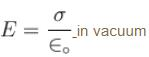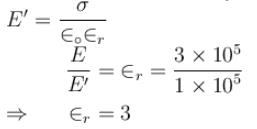Surface density of induced charges,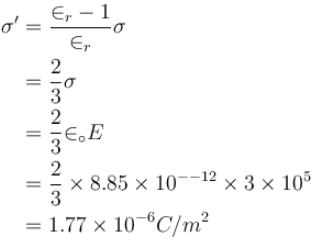The correct answer is: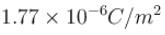QUESTION: 3

### Polarization in a dielectric on application of electric field is

Solution:

The correct answer is: Displacement / separation of opposite charge centers

QUESTION: 4

The outer sphere of spherical capacitor is earthed. For increasing its capacitance.

Solution:

The correct answer is: dielectric material is filled between the two spheres

QUESTION: 5

The relation between bound charge density ρb to free charge (extraneous charge) ρf is given by

Solution:

The correct answer is: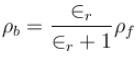QUESTION: 6

If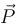is the polarization vector and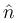is the unit vector normal to the surface then a polarized piece of a dielectric can be regarded as equivalent to

Solution:

The correct answer is: charges of a volume density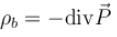and surface charges of density ρb is given by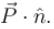QUESTION: 7

The susceptibility of a polar dielectric is temperature dependent because

Solution:

The correct answer is: They are necessarily anisotropic in a nature

QUESTION: 8

At the boundary or interface between two dielectric media the vector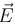and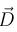are such that

Solution:

The correct answer is: The tangential component ofand the normal component ofis same on each side of the boundary. The latter condition is however, valid only in the absence of any free charges on the interface

QUESTION: 9

In a dielectric conductor boundary (interface). The tangential component of electric field is

Solution: